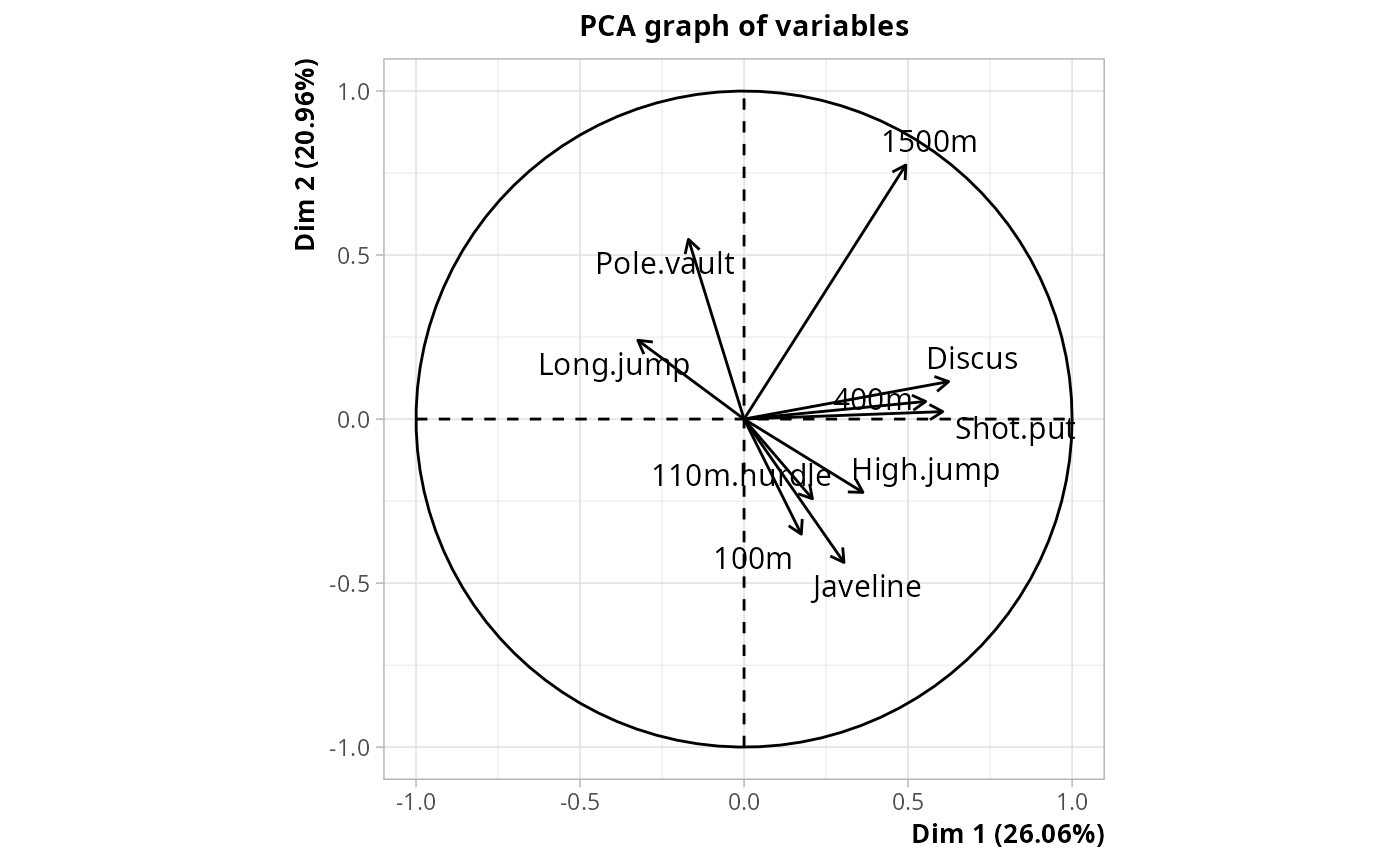Principal Component Analysis with Orthogonal Instrumental Variables

PCAoiv(X, Z, row.w = NULL, ncp = 5)

## Arguments

X

data frame with only numeric variables

Z

data frame of instrumental variables to be "partialled out"", which can be numeric or factors. It must have the same number of rows as X.

row.w

Numeric vector of row weights. If NULL (default), a vector of 1 for uniform row weights is used.

ncp

number of dimensions kept in the results (by default 5)

## Details

Principal Component Analysis with Orthogonal Instrumental Variables consists in two steps : 1. Computation of one linear regression for each variable in X, with this variable as response and all variables in Z as explanatory variables. 2. Principal Component Analysis of the set of residuals from the regressions in 1.

## Value

An object of class PCA from FactoMineR package, and an additional item :

ratio

the share of inertia not explained by the instrumental variables

.

## Author

Nicolas Robette

wcPCA, wcMCA, MCAoiv
library(FactoMineR)